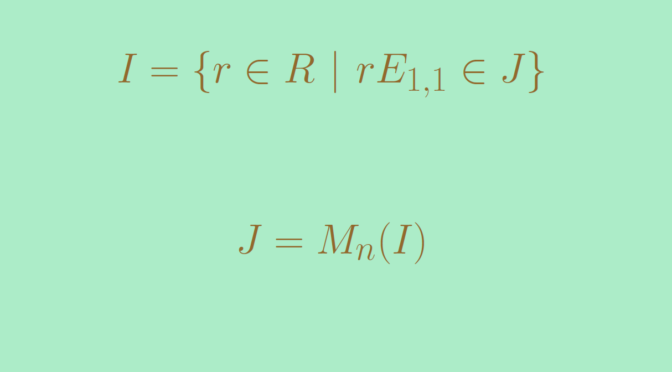# A simple ring which is not a division ring

Let’s recall that a simple ring is a non-zero ring that has no two-sided ideal besides the zero ideal and itself. A division ring is a simple ring. Is the converse true? The answer is negative and we provide here a counterexample of a simple ring which is not a division ring.

We prove that for $$n \ge 1$$ the matrix ring $$M_n(F)$$ of $$n \times n$$ matrices over a field $$F$$ is simple. $$M_n(F)$$ is obviously not a division ring as the matrix with $$1$$ at position $$(1,1)$$ and $$0$$ elsewhere is not invertible.

Let’s prove first following lemma.

Lemma. For a commutative ring $$R$$, every two-sided ideal of $$M_n(R)$$ is of the form $$M_n(I)$$ for a two-sided ideal $$I$$ of $$R$$.

Let $$J \subseteq M_n(R)$$ be a two-sided ideal. Let $$E_{i,j}$$ be the matrix with $$1$$ at position $$(i,j)$$ and $$0$$ elsewhere. Recall that matrices $$E_{i,j}$$ satisfy the relations
\begin{gather*}
E_{i,j}E_{j,k} = E_{i,k}\\
E_{i,j}E_{l,k} = 0 \text{ for } j \neq l
\end{gather*} and for a matrix $$A=(a_{i,j})$$, we have $E_{m,i}AE_{j,k}= a_{i,j}E_{m,k}$ Now let $I=\{r \in R \ | \ r E_{1,1} \in J\}$ $$I$$ is a two-sided ideal of $$R$$. Indeed, let $$a \in R$$ and $$r \in I$$. We have $$(a E_{1,1})(r E_{1,1}) = ar E_{1,1}$$ hence $$ar \in I$$. Similarly, $$ra \in I$$.

For any matrix $$A \in J$$ we have $a_{i,j} E_{1,1} = E_{1,i} A E_{j,1}$ As $$J$$ is an ideal, the right hand side belongs to $$J$$ and hence $$a_{i,j} \in I$$. It follows that $$J \subseteq M_n(I)$$. Conversely an element $$A \in M_n(I)$$ is a sum of elements of the form $$r E_{i,j}$$ with $$r \in I$$. The equality $$E_{i,1} (r E_{1,1} ) E_{1,j} = r E_{i,j}$$ implies that $$r E_{i,j} \in J$$ for $$r \in I$$. Therefore $$A \in J$$ and we have proven $$J=M_n(I)$$.

For a field $$F$$, we can easily conclude with the lemma that $$M_n(F)$$ is a simple ring as the only ideals of a field $$F$$ are $$\{0\}$$ and the field $$F$$ itself.